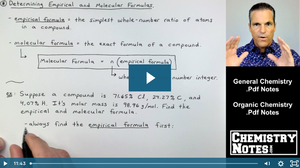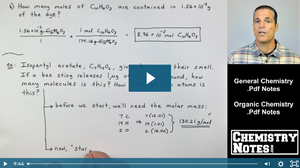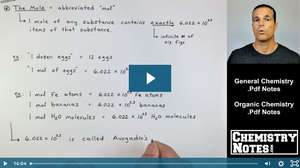# Chemistry Crash Course | Chemistry Help — SECTION 3 - Chemical Quantities and Stoichiometry

## S3E3 - How to Find the Empirical Formula and Molecular FormulaEmpirical Formula Calculations, How to Find the Empirical Formula, and How to Find the Molecular Formula When Given Empirical Formula Examples and Problems.

## S3E2 - How to Find Molar Mass, Grams-to-Moles, and Grams-to-MoleculesGrams to Moles Calculations, Grams to Molecules Conversions, How to Find Molar Mass, and How to Calculate Mass Percent, or Percent Composition, of a Compound.